Saturday 2nd July 2022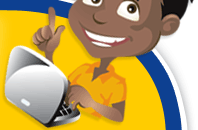# Classroom Key Stage 2 / KS2 - Maths Activities & Resources

## Welcome to the Maths section of the Key Stage 2 classroom. Within KS2 Maths you will find online @school assessment tests that will help children to enhance their learning and revise the subject of Maths at Key Stage 2 level.

•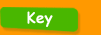•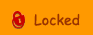•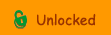••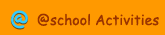•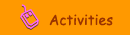•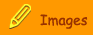###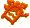Using a Calculator

Area Calculations - Use the online calculator to solve these area calculations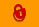Calculator Clipart - Clipart of Calculators.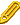Calculator Problems - Use the online calculator to solve these problems.Online Calculator - Use the @school calculator to do your calculationsPercentage Calculations - Use the online calculator to solve these percentage problems.Using a Calculator - Interactive test about using a calculator.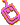Using a Calculator - Interactive activity to use a calculatorUsing a Calculator  Checking - Interactive activity to use a calculator to check calculationsUsing a Calculator  Checking 2 - Interactive activity to use a calculator to check calculationsUsing a Calculator  Errors - Interactive activity to use a calculatorUsing a Calculator  Memory - Interactive activity to use the calculator memoryUsing a Calculator  Memory 2 - Interactive activity to use a calculator memoryUsing a Calculator - Money - Interactive activity to use a calculatorUsing a Calculator  Two or More Step Operations - Interactive activity to use a calculator